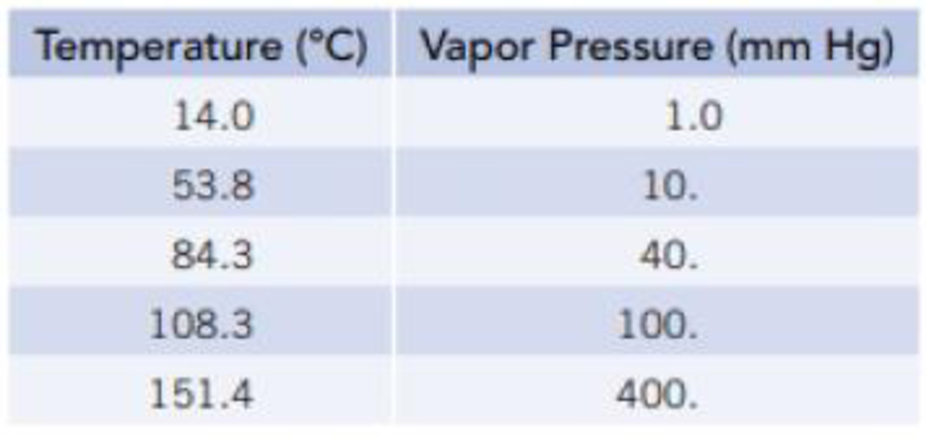Chapter 11, Problem 38GQ

Chapter
Section
Textbook Problem

The following data are the equilibrium vapor pressure of limonene, C10H16, at various temperatures. (Limonene is used as a scent in commercial products.)(a) Plot these data as ln P versus 1/T so that you have a graph resembling the one in Figure 11.13. (b) At what temperature does the liquid have an equilibrium vapor pressure of 250 mm Hg? At what temperature is it 650 mm Hg? (c) What is the normal boiling point of limonene? (d) Calculate the molar enthalpy of vaporization for limonene using the Clausius-Clapeyron equation.

(a)

Interpretation Introduction

Interpretation:

The graph of lnPversus1/T using the given data has to be plotted.

Concept Introduction:

Clausius-Clapeyron equation:

lnP=(ΔvapH0RT)+C

From this relationship we can calculate the molar enthalpy of vaporization by knowing the corresponding temperature and pressure values.

If we have pressures at two different temperatures, then enthalpy of vaporization can be calculated by

lnP2p1=-ΔvapH0R[1T2-1T1]

Explanation

The graph of lnP versus 1/T is plotted using given data.

Given:

Temperature(K)VapourPressure(mmHg)2871326.810357.340381.3100424

(b)

Interpretation Introduction

Interpretation:

The temperature at which the liquid has vapour pressures of 250mmHg and 650mmHg has to be determined.

Concept Introduction:

Clausius-Clapeyron equation:

lnP=(ΔvapH0RT)+C

From this relationship we can calculate the molar enthalpy of vaporization by knowing the corresponding temperature and pressure values.

If we have pressures at two different temperatures, then enthalpy of vaporization can be calculated by

lnP2p1=-ΔvapH0R[1T2-1T1]

(c)

Interpretation Introduction

Interpretation:

The normal boiling point of limonene has to be determined.

Concept Introduction:

Clausius-Clapeyron equation:

lnP=(ΔvapH0RT)+C

From this relationship we can calculate the molar enthalpy of vaporization by knowing the corresponding temperature and pressure values.

If we have pressures at two different temperatures, then enthalpy of vaporization can be calculated by

lnP2p1=-ΔvapH0R[1T2-1T1]

Boiling point of a liquid: The temperature at which external pressure and vapour pressure of the liquid become same.

Normal boiling point: When the external pressure is 760mmHg we can call it as normal boiling point.

(d)

Interpretation Introduction

Interpretation:

The molar enthalpy of vaporization has to be determined

Concept Introduction:

Clausius-Clapeyron equation:

lnP=(ΔvapH0RT)+C

From this relationship we can calculate the molar enthalpy of vaporization by knowing the corresponding temperature and pressure values.

If we have pressures at two different temperatures, then enthalpy of vaporization can be calculated by

lnP2p1=-ΔvapH0R[1T2-1T1]

Molar enthalpy of vaporization: The energy required to convert liquid to gas of 1mol of a substance is called molar enthalpy of vaporization

Still sussing out bartleby?

Check out a sample textbook solution.

See a sample solution

The Solution to Your Study Problems

Bartleby provides explanations to thousands of textbook problems written by our experts, many with advanced degrees!

Get Started

Find more solutions based on key concepts